#Function Repository Resource:

# LookupCases

Look up all values of a key in a nested association

Contributed by: Gerli Jõgeva
 ResourceFunction["LookupCases"][expr,key] gives a list of all values associated with key in the expression expr. ResourceFunction["LookupCases"][expr,key,levelspec] gives a list of all values associated with key in the expression expr on levels specified by levelspec. ResourceFunction["LookupCases"][expr,key,levelspec,n] gives the first n values in expr associated with key. ResourceFunction["LookupCases"][key] represents an operator form of ResourceFunction["LookupCases"] that can be applied to an expression.

## Details and Options

LookupCases uses standard level specifications:
 n levels 1 through n levels 1 through Infinity { n } level n only { n , m } levels n through m levels 0 through Infinity
The default value for levelspec in ResourceFunction["LookupCases"] is {0,Infinity} or All.
The default value for the number of values n is Infinity.
ResourceFunction["LookupCases"] traverses the parts of expr in a depth-first order, with leaves visited before roots.

## Examples

### Basic Examples

LookupCases allows you to look up the values of a key anywhere in the structure, without knowing its location:

 In:=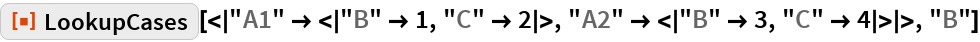Out=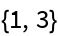Use the operator form:

 In:=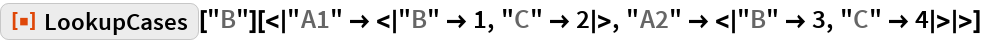Out=### Scope

An empty list is returned when no matches are found:

 In:=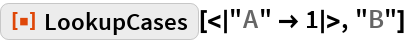Out=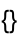Looking up a delayed value causes the value to evaluate:

 In:=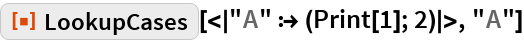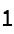Out=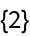If the delayed value is in a non-matching key, evaluation does not occur:

 In:=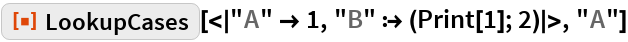Out=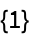Do not look up values on the top-level association:

 In:=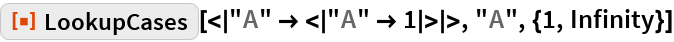Out=Only return the first match:

 In:=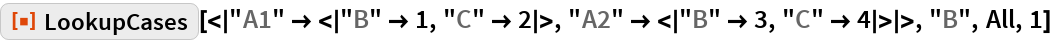Out=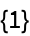LookupCases also finds the value from a list of rules:

 In:=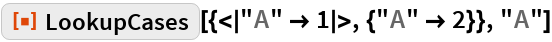Out=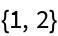If the list contains other items besides rules, the match is not found:

 In:=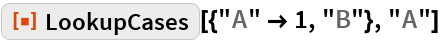Out=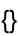In:=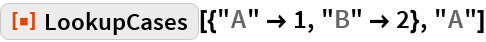Out=Use a pattern for the key:

 In:=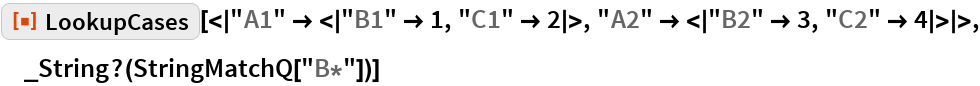Out=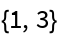### Possible Issues

In a nested structure with the same key, both values are returned:

 In:=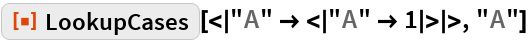Out=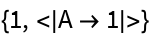## Requirements

Wolfram Language 11.3 (March 2018) or above